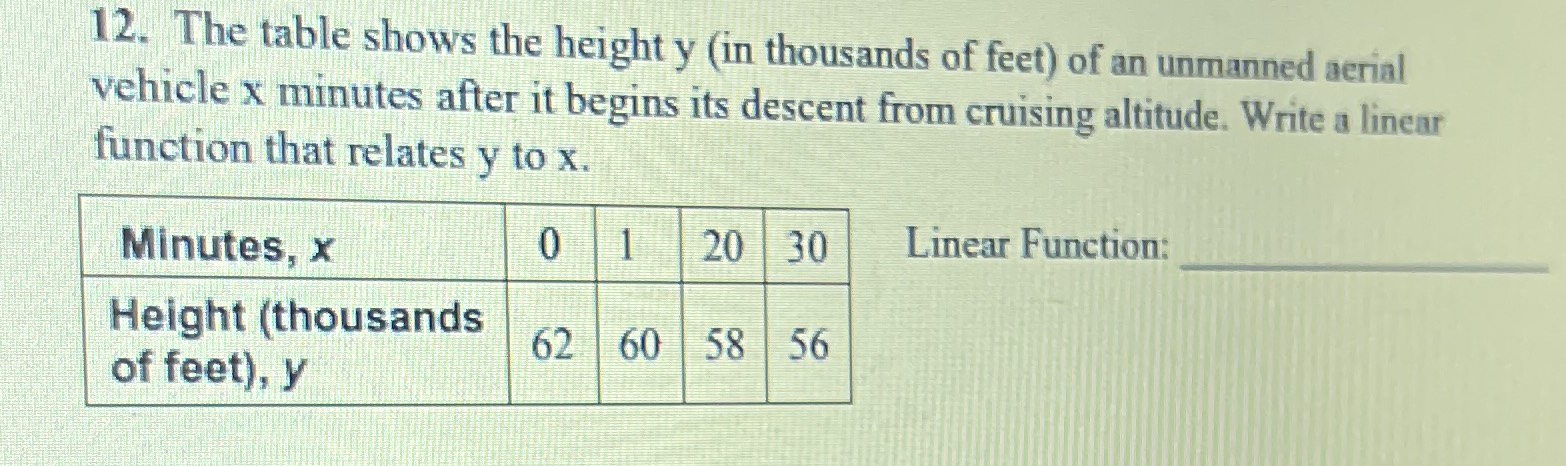### ¿Todavía tienes preguntas de matemáticas?

Pregunte a nuestros tutores expertos
Algebra
Pregunta12. The table shows the height $$y$$ (in thousands of feet) of an unmanned aerial vehicle $$x$$ minutes after it begins its descent from cruising altitude. Write a linear function that relates $$y$$ to $$x$$ .

Linear Function: